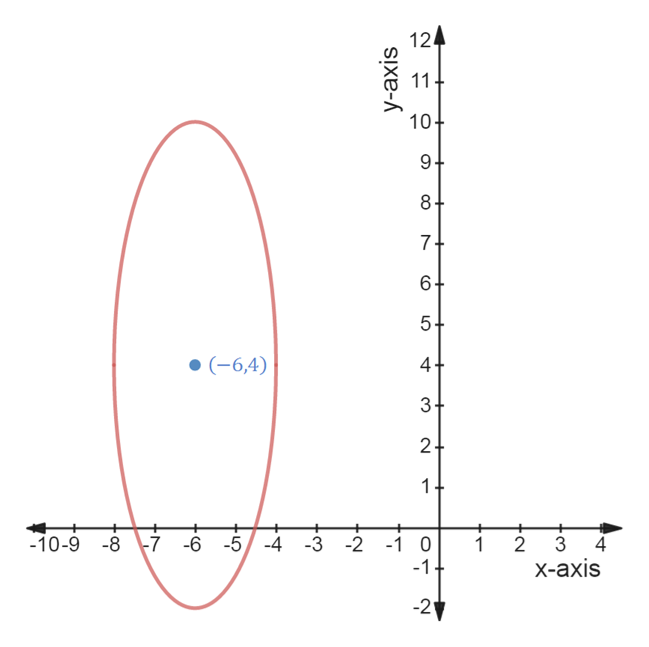# 28. An ellipse has the equation (x+6)2 /4 + (y− 4)2/36=1(a) Is the major axis horizontal or vertical?(b) Find the exact values of the foci of the ellipse. Show work.

Question
2 views

28. An ellipse has the equation (x+6)2 /4 + (y− 4)2/36=1
(a) Is the major axis horizontal or vertical?
(b) Find the exact values of the foci of the ellipse. Show work.

check_circle

Step 1

Given:

Step 2

General equation of ellipse is given by

Step 3

Graph of ell...help_outlineImage Transcriptionclose124 10+ 9 8+ 74 5+ (-6,4) 4+ 3+ 2+ 1+ + + + + -10-9 -8 Ť -6 -5-4 -3 -2 -1 0 1 2 -1+ X-ахis -2 CO LO 11 y-axis fullscreen

### Want to see the full answer?

See Solution

#### Want to see this answer and more?

Solutions are written by subject experts who are available 24/7. Questions are typically answered within 1 hour.*

See Solution
*Response times may vary by subject and question.
Tagged in

### Other